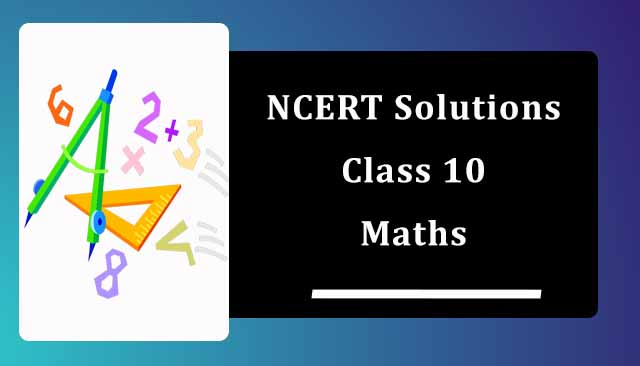# NCERT Solutions for Class 10 Maths

NCERT Solutions for Class 10 Maths is arranged here chapterwise and exercise wise which you can use according to own comfort. There are total 15 chapter is the Class 10 Maths textbook which are very important for the purpose of examinations. Stackgully has provided accurate and detailed NCERT Solutions of every problem so you can easily understand the concept behind. These NCERT Solutions for Class 10 Maths will improve your logical skills and at the same time help you in scoring good marks in the examinations. While solving questions if you find any proble hard, then you can refer from these solutions easily.You can also check NCERT Solutions for Class 10 Science which are very important for every student.

## NCERT Solutions for Class 10 Maths

Real Numbers Class 10 Ex 1.1
Real Numbers Class 10 Ex 1.2
Real Numbers Class 10 Ex 1.3
Real Numbers Class 10 Ex 1.4

Polynomials Class 10 Ex 2.1
Polynomials Class 10 Ex 2.2
Polynomials Class 10 Ex 2.3
Polynomials Class 10 Ex 2.4

NCERT Solutions of Chapter 3 Pair of Linear Equations in Two Variables

Pair of Linear Equations in Two Variables Class 10 Ex 3.1
Pair of Linear Equations in Two Variables Class 10 Ex 3.2
Pair of Linear Equations in Two Variables Class 10 Ex 3.3
Pair of Linear Equations in Two Variables Class 10 Ex 3.4
Pair of Linear Equations in Two Variables Class 10 Ex 3.5
Pair of Linear Equations in Two Variables Class 10 Ex 3.6
Pair of Linear Equations in Two Variables Class 10 Ex 3.7

NCERT Solutions of Chapter 4 Quadratic Equations

Quadratic Equations Class 10 Ex 4.1
Quadratic Equations Class 10 Ex 4.2
Quadratic Equations Class 10 Ex 4.3
Quadratic Equations Class 10 Ex 4.4

NCERT Solutions of Chapter 5 Arithmetic Progressions

Arithmetic Progressions Class 10 Ex 5.1
Arithmetic Progressions Class 10 Ex 5.2
Arithmetic Progressions Class 10 Ex 5.3
Arithmetic Progressions Class 10 Ex 5.4

NCERT Solutions of Chapter 6 Triangles

Triangles Class 10 Ex 6.1
Triangles Class 10 Ex 6.2
Triangles Class 10 Ex 6.3
Triangles Class 10 Ex 6.4
Triangles Class 10 Ex 6.5
Triangles Class 10 Ex 6.6

NCERT Solutions of Chapter 7 Coordinate Geometry

Coordinate Geometry Class 10 Ex 7.1
Coordinate Geometry Class 10 Ex 7.2
Coordinate Geometry Class 10 Ex 7.3
Coordinate Geometry Class 10 Ex 7.4

NCERT Solutions of Chapter 8 Introduction to Trigonometry

Introduction to Trigonometry Class 10 Ex 8.1
Introduction to Trigonometry Class 10 Ex 8.2
Introduction to Trigonometry Class 10 Ex 8.3
Introduction to Trigonometry Class 10 Ex 8.4

NCERT Solutions of Chapter 9 Some Applications of Trigonometry

Some Applications of Trigonometry Class 10 Ex 9.1

NCERT Solutions of Chapter 10 Circles

Circles Class 10 Ex 10.1
Circles Class 10 Ex 10.2
Chapter 11 Constructions

NCERT Solutions of Constructions Class 10 Ex 11.1

Constructions Class 10 Ex 11.2

NCERT Solutions of Chapter 12 Areas Related to Circles

Areas Related to Circles Class 10 Ex 12.1
Areas Related to Circles Class 10 Ex 12.2
Areas Related to Circles Class 10 Ex 12.3

NCERT Solutions of Chapter 13 Surface Areas and Volumes

Surface Areas and Volumes Class 10 Ex 13.1
Surface Areas and Volumes Class 10 Ex 13.2
Surface Areas and Volumes Class 10 Ex 13.3
Surface Areas and Volumes Class 10 Ex 13.4
Surface Areas and Volumes Class 10 Ex 13.5

NCERT Solutions of Chapter 14 Statistics

Statistics Class 10 Ex 14.1
Statistics Class 10 Ex 14.2
Statistics Class 10 Ex 14.3
Statistics Class 10 Ex 14.4

NCERT Solutions of Chapter 15 Probability

Probability Class 10 Ex 15.1
Probability Class 10 Ex 15.2